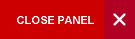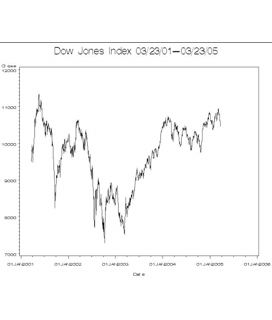# Department of Mathematics## Research

At present the most developed part of the research activity of the Department of Mathematics can be characterized as “Mathematical Modelling”. It comprises such fields as stochastic analysis, physiological fluid dynamics, and mathematical physics. Moreover, starting with the semester 2010-11 the Department of Mathematics will launch a new Double Major in Mathematics and Modelling Processes. In addition  the department is currently engaged with UWI (St. Augustine, Trinidad) in a cross-campus collaboration in Symmetries of Differential Equations. The group presently includes Prof. Bhatt (St. Augustine) and Prof. Rodkina (Mona), who are jointly supervising a Ph.D. student based at Mona. Recently, the Department of Mathematics begun to be involved into the research in the area of in Mathematical Education, and we expect the formation of the research group soon.

The following research areas are currently active in the Mathematics Department

Stochastic Differential and Difference EquationsStochastic equations are used to model real-world systems that are subject to interference in the form of randomexternal perturbations or feedback noise. This interference can have a dramatic qualitative effect on these systems and should therefore be included in any analysis of their behaviour. For example population models may be designedto reflect the unpredictable effects of disease and environment, as well as classical predator-prey, birth-death dynamics.

The Stochastic Dynamics group presently includes Prof. Rodkina, Dr. Kelly, a PhD student and several internationalcollaborators. You can download the poster of the Stochastic Dynamics group for the research day held at the Universityof West Indies in January 2010 here and in January 2015 here.

Physiological Fluid DynamicsPhysiological fluid dynamics involves the study of idealized model problems (either experimental or theoretical) that characterize the key features of flows in the body. Being an interdisciplinary subject, its development is due to the close interaction between engineers, mathematicians, physicists, biologists and physiologists. Mathematical models have proved to be powerful tools for understanding not only the normal, but also the pathological conditions of the physiological systems. The information gained from such studies has contributed to the improved efficiency
• in the diagnosis of various arterial diseases,
• the appraisal of newly found treatment procedures,
• designing of artificial organs.

Some of the models that have gained lot of interest to understand the physiological systems are

1. Modelling of flow in stenosed arteries;
2. Modelling of flow in catheterized vessels;
3. Modelling of Dispersion/Transport phenomena in cardiovascular system;
4. Modeling Peristaltic transport in physiological fluids.
The objective of these models is to understand the physiological process through mathematical models and fluid dynamic principles and the results obtained from these mathematical models compare with the experimental or measured results. This research is lead by Dr. Ponakala and include a PhD student and several international collaborators.

Mathematical physicsMathematical physics is a discipline concerned with the application of mathematics to problems in physics and with the development of mathematical methods suitable for such applications and for the formulations of physical theories. The term mathematical physics denotes research aimed at studying and solving problems inspired by physics within a mathematically rigorous framework. For this reason mathematical physics covers a very broad area of topics having the common feature that they blend pure mathematics and physics. Research activity is mainly focussed on
1. General Relativity,Differential Geometry, and Noncommutative Geometry inspired solutions of Einstein field equations;
2. Quantum Field Theory in curved space times;
3. Spectral theory of Schrödinger, Dirac and Klein-Gordon operators;
4. Quantum Gravity:

and is lead by Dr. Batic. This is a collaboration with Dr. Piero Nicolini (University of Frankfurt), Dr. Marek Nowakowski (Universidad de los Andes),Dr. Monika Winklmeier (Universidad de los Andes) and Prof. Gian Michele Graf(ETH).

Top of Page